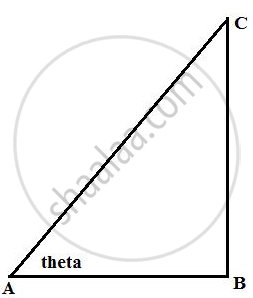# In Right-angled Triangle Abc; B = 90°. Find the Magnitude of Angle A, If: Ab is √ 3 Times of Bc. - Mathematics

Sum

In right-angled triangle ABC; ∠ B = 90°. Find the magnitude of angle A, if: AB is √3 times of BC.

#### Solution

Consider the figureHere AB is sqrt(3) times of BC means

"AB"/"BC" = sqrt(3)

cot θ = cot 30°

θ = 30°

Concept: Solution of Right Triangles
Is there an error in this question or solution?

#### APPEARS IN

Selina Concise Mathematics Class 9 ICSE
Chapter 24 Solution of Right Triangles [Simple 2-D Problems Involving One Right-angled Triangle]
Exercise 24 | Q 14.1 | Page 304